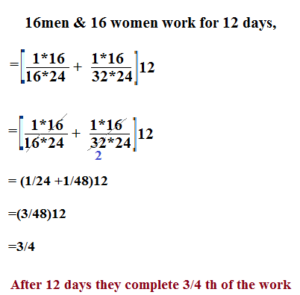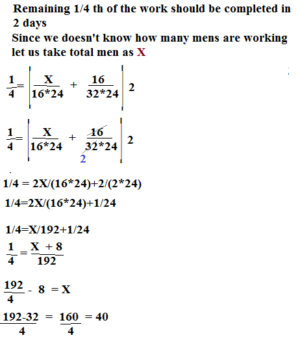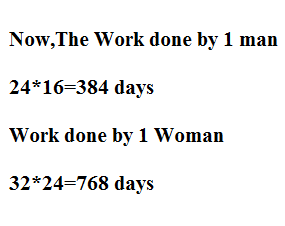# SHORTCUTS TO SOLVE TIME & WORK:HOW TO SERIES

Hi Bankersdaily Aspirants,

There is exactly 10 days for our IBPS RRB Prelims Examination,this is the most important time for our career & from our side we are giving full support for  all the aspirants.For the Beginners we had started Learn Series & it went well without any hurdle .If you are beginner & you hadn’t look into Learn Series,Check now because we had discussed all the basic concepts in both Aptitude & Reasoning Section

### INTRODUCTION:

Friends today we came with,new problems on Time & Work,As you all think Time & Work is not that much tough if we know the shortcuts and the relationship between the terms in time & work.We had already discussed Basic Concepts in Time & Work before looking into Problems Let us look into Basic Concept in Time & Work.

1)24 men can complete the piece of work in 16 days at the same time 32 men can complete the same work in 24 days.If 16 men & 16 women work together for 12 days.Find how men should be added if the remaining work should be completed in 2 days?

EXPLANATION

Given that,

24 Men can Complete the work in 16 days

32 Men can Complete the work in 24 days

16 Men + 16 Women work together for 12 days,### Remaining 1/4 th of the work is to be completed with the help of additional menFrom this we found that the number of Men=40

But initially there are 16 men working So Additional Men required is 40-16=24 Men

Therefore,we need 24 Men additionally to complete the work.

### Do you think Can we crack the exam if we use this method to solve these kinds of Problems?

Then My Answer would be BIG NO,It is waste of time since we have to answer 100 questions in 1 hour,So let us look the Alternate Method to solve these kinds of Problems,This is Quite Easy compared to the above method,

We Just want to Know What is Efficiency?
,As you all know

Efficiency is Work done by an individual or a group in 1 day

Efficiency=Work done/Number of Days Taken

EXPLANATION FOR ALTERNATE METHOD

What is Given in the Question?

24 Men can Complete the work in 16 days

The same work,

32Women can Complete the work in 24 daysTotal Work=LCM(384,768)=768

Efficiency of Men=768/384=2 work/day

Efficiency of Women=768/768=1 work/day

Therefore 16 Men +16 Women work for 12 days

Then they  would complete,

=(16*2+16*1)*12

=(32+16)*12

=48*12

=576 Work

So when they work for 12 days they would complete 576 Work

Remaining Work=768-576=192 work

In this 192 Work 16 Women will complete 32 Work(Since 16 women can Complete 16 work per day therefore for 2 days they will complete 16*2=32 work)

Therefore Remaining Work=192-32=160work

160 has to be completed by Men alone

=160/2*2(2 days and Efficiency of 1 man =2,So only we had written 2*2)

=160/4

=40 Men

There is already 16 Men So we need 40-16-24 Men

Friends I think you found my alternate Method as easier & I just Explained in detail because you just want to understand the concept or else this sum can be solved in just 3 steps.You can also solve this problem in just three steps if you practice well,So Practice this sum.